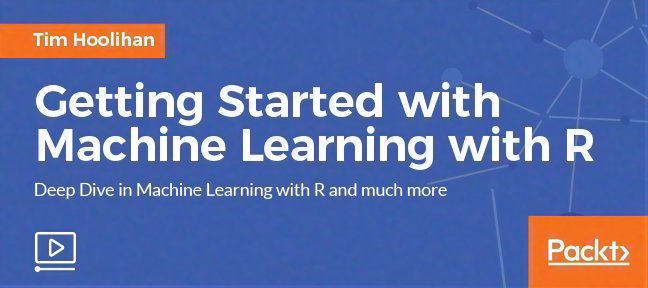# Video Tutorial Getting Started with Machine Learning with Rwith R, Data Science

• Course duration : 1h20m
• 30 days money back guarantee
• Source files included
• Discover why you should learn on Tuto.comA fast-paced guide to getting started with the Machine Learning with R.

## About Getting Started with Machine Learning with R video course

• Dive into the essential know-how of Machine learning with R.
• Practical, real-world examples to help you master the various stages of machine learning using the R language.
• Look into important machine learning concepts such as linear and logistic regression, data scaling, and cross-validation.

### Video course In Detail

Machine learning is a subfield of computer science that gives computers the ability to learn without being explicitly programmed. It explores the study and construction of algorithms that can learn from, and make predictions on, data. The R language is widely used among statisticians and data miners to develop statistical software and perform data analysis. Machine Learning is a growing field that focuses on teaching computers to do work that was traditionally reserved for humans; it is a cross-functional domain that uses concepts from statistics, math, software engineering, and more.

In this video tutorial you will start by organizing your data and then predicting it. Then you will work through various examples.

• The first example will demonstrate (using linear regression) predicting the murder arrest rate based on arrest data for a given State. Here you will explore R Studio and libraries, how to apply linear regression, how to score test sets, and plotting test results on a Cartesian plane.
• Then the next example will use logistic regression to predict for a classification problem on breast cancer: forecasting insurance types based on medical treatment. This example demonstrate labeling and scaling data, how cross-validation works, and how to apply Logistic regression.
• Finally you will move on the next example—automobile classification—where you will use the caret package in R to simplify some of these steps.

By the end of this video course, you will have mastered preparing data and the tools involved: regression and classification. Additionally, you will have learned to make predictions on new observations.

• Linear Regression: Predicting Arrests
• The Course Overview free 00:01:56
• Exploring the US Arrests Dataset 00:05:02
• Creating Test and Train Datasets 00:03:50
• Creating a Linear Regression Model 00:03:47
• Scoring on the Test Set 00:05:29
• Plotting the Test Results 00:04:15
• Classification
• EDA: mtcars 00:03:27
• Working with Factors 00:04:28
• Scaling Data 00:04:10
• Creating a Classification Model 00:02:30
• Precision, Recall, and F-Score 00:05:19
• Caret
• Introduction to Caret 00:02:33
• EDA and Preprocessing 00:10:59
• Preparing Test and Train Datasets 00:03:08
• Creating a Model 00:03:08
• Cross Validation 00:03:33
• F-Score 00:06:00

## Instructor : Packt

Packt has published 46 tutorials and has sold 8 coursess. See others courses from Packt

• With which software version is this tutorial compatible with?
R
• What is the required level to follow this tutorial ?
intermediate